Nonequispaced fast Fourier transform
Interpolation
iNFFT

# Interpolation

Let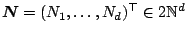. For given samples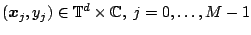, the index set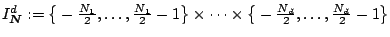, of frequencies, we construct a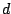-variate trigonometric polynomial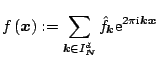such that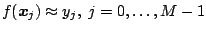. Turning this into matrix vector notation, we aim to solve the system of linear equations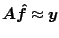(1)

for the unknown vector of Fourier coefficients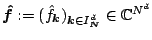. We denote the vector of the given sample values by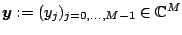and the nonequispaced Fourier matrix by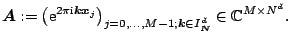We focus on the under-determined and consistent linear system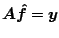, i.e., we expect to interpolate the given data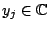,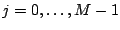, exactly.

In particular, we incorporate damping factors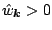,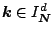, and consider the optimal interpolation problem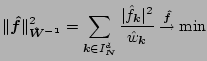subject to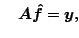(2)

where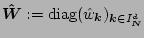.

This library of C functions computes approximations of (2) with the CGNE method.

The algorithms are implemented by Stefan Kunis in ./solver. Related paper areKunis, S. and Potts, D.
Stability Results for Scattered Data Interpolation by Trigonometric Polynomials.
SIAM J. Sci. Comput. 29, 1403 - 1419,   (full paper ps, pdf)   2007